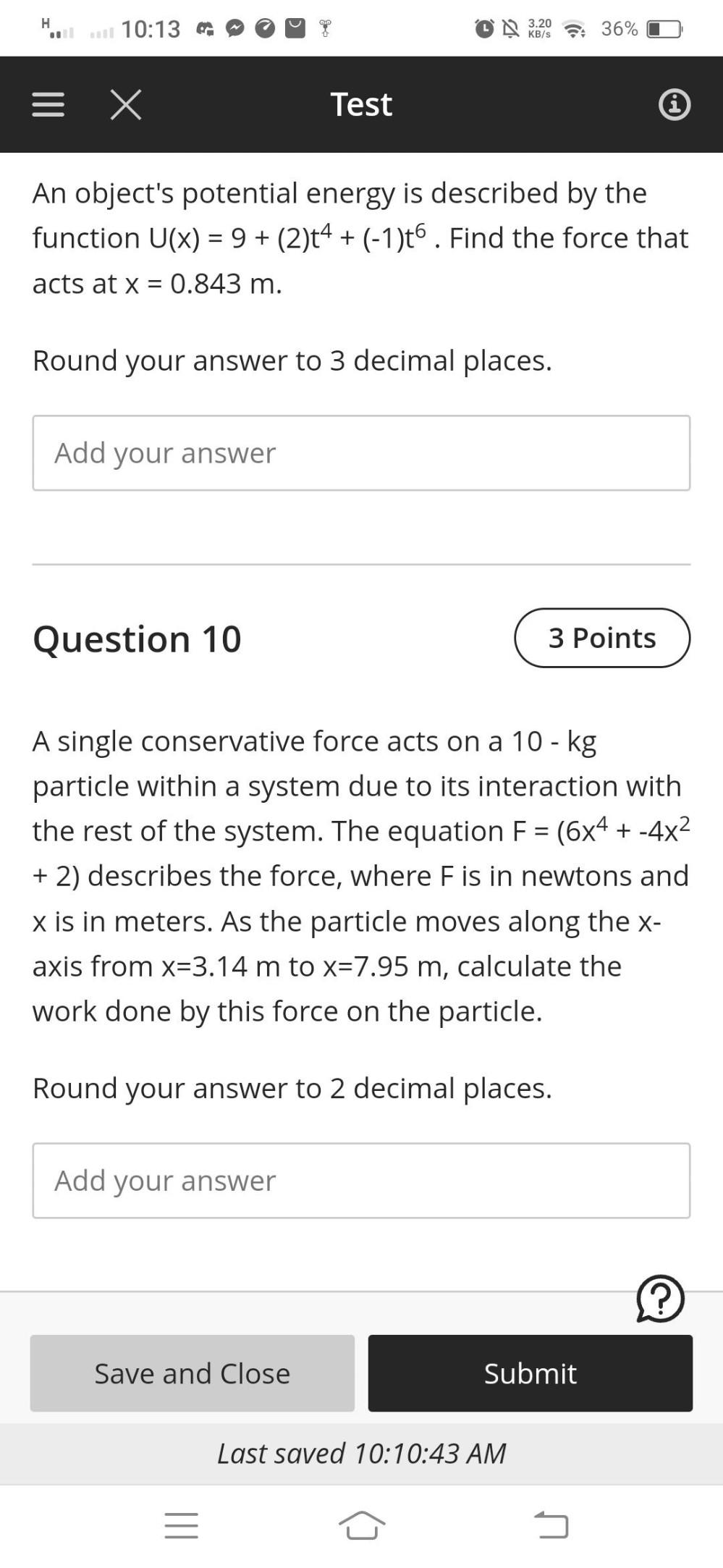Question:

# H 10:13 3:20 36% KB/S = x Test An object's potential energy is described by the function U(x) = 9+ (2)t4 + (-1)06. Find the forcH 10:13 3:20 36% KB/S = x Test An object's potential energy is described by the function U(x) = 9+ (2)t4 + (-1)06. Find the force that acts at x = 0.843 m. = Round your answer to 3 decimal places. Add your answer Question 10 3 Points = A single conservative force acts on a 10-kg particle within a system due to its interaction with the rest of the system. The equation F = (6x4 + -4x2 + 2) describes the force, where F is in newtons and x is in meters. As the particle moves along the x- axis from x=3.14 m to x=7.95 m, calculate the work done by this force on the particle. Round your answer to 2 decimal places. Add your answer ? Save and Close Submit Last saved 10:10:43 AM 5